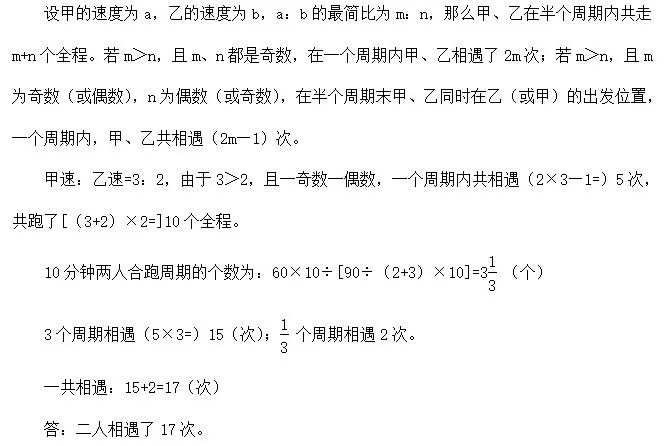# 小学数学天天练|全年级覆盖~2019.8.21

【题目】

【答案】

【答 案】：36-7=29（个）

【解 答】：这道题是三个数相加，通过观察不难发现，5和45先算可以凑成50，这样计算起来比较容易

=37+（5+45）

=37+50

=87

【答 案】：用去：25-14=11（千克）水重：11×2=22（千克）

【解 析】：把81分解因数为9×9是为了下一步提出公因数9。

【答 案】：

81+991×9

=9×9+991×9

=(9+991)×9

=1000×9

=9000